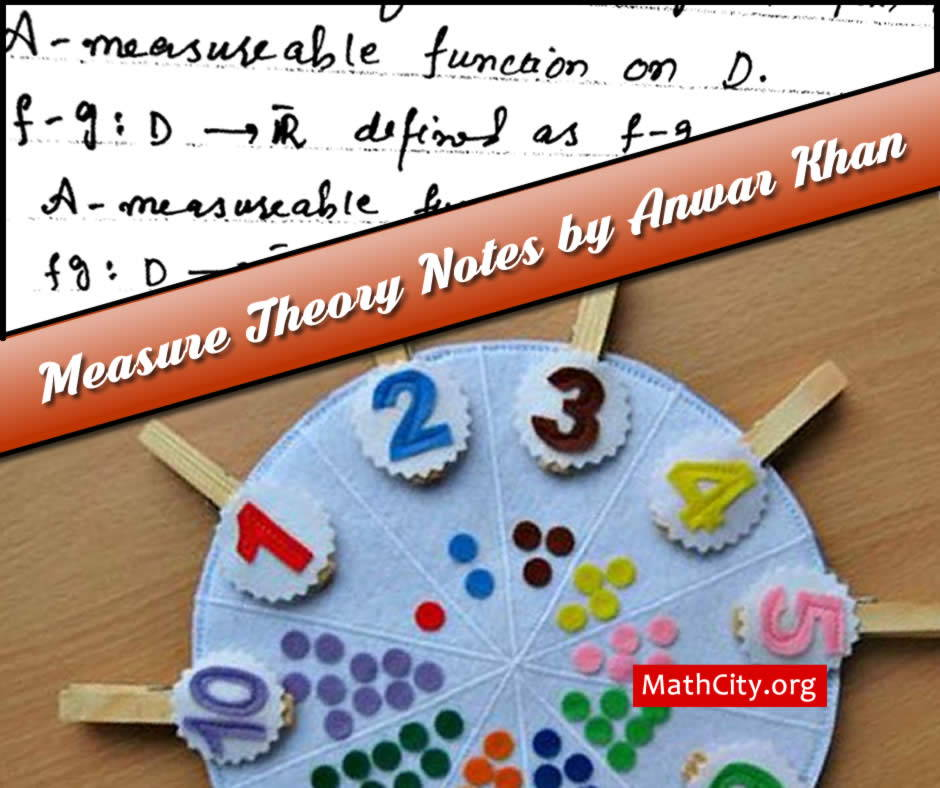# Measure Theory Notes by Anwar KhanMeasure theory is a branch of mathematics concerned with the concept of “measure,” which is a method of assigning a numerical value to specific sets. The concepts of length, area, and volume are generalised via measurements to more abstract environments, such as infinite-dimensional spaces and areas that cannot be seen.

In measure theory, a measure is a function that assigns a non-negative integer to subsets of a given set. Additionally, the function must meet a number of requirements, such as being countably additive, which requires that the measure of a countable union of disjoint sets equal the sum of those measures.

Measure theory has applications in many areas of mathematics, including analysis, probability theory, and topology. The creation of the Lebesgue integral, a more versatile and effective definition of integration than the Riemann integral, also relies heavily on measure theory.

These are the handwritten notes of measure theory by Anwar Khan, PhD. These notes are useful for covering measurement theory papers at the BS and MS levels. For sending us these notes, Anwar Khan, PhD has our sincere gratitude.

• Name: Measure Theory: Notes
• Provider: Mr. Anwar Khan
• Pages: 169 pages
• Format: PDF
• Size: 7.8 MB
• Algebra on $X$
• Sigma Algebra i.e. $\sigma-$algebra on $X$
• Trivial $\sigma-$algebra; Largest $\sigma-$algebra
• Increasin & sequence of sets
• Decreasing sequence of sets
• Define $\lim\limits_{k\to \infty} \sup A_k$ and $\lim\limits_{k\to \infty} \inf A_k$
• Smallest $\sigma-$algebra
• Borel set & Borel $\sigma-$algebra
• $G_\sigma-$set; $F_\sigma-$set
• Set of extended real numbers; Set function; Properties of set function
• Measure
• Finite measure; $\sigma-$finite measure
• Monotone convergence theorem
• Measurable space and measure space; Finite measure space; $\sigma-$finite measure space; $\mathcal{A}-$measurable set
• $\sigma-$finite set
• Null set
• Complete $\sigma-$algebra; Complete
• measure space; Outer measure
• $\mu^*-$measurable set
• Lebesgue outer measure
• Lebesgue measurable set or $\mu^*-$measurable set; Lebesgue $\sigma-$algebra; Lebesgue measurable space
• Lebesgue measure space
• Dense sub set of $X$
• Translation of a set; Dielation of a set
• Translation invarient
• Translation of $E$ mod 1
• Measurable function
• Characteristic function
• Almost every where property; Equal almost every where
• Limit inferior and limit superior of real value sequence
• Sequence of $\mathcal{A}-$measurable functions & its limits & their properties
• Larger & smaller of two function; Positive part of $f$; Negative part of $f$; Absolute function of $f$
• Limit existence almost every where
• Step function
• Riemann integral
• Simple function; Canonical representation of simple function
• Lebesgue integral of simple function
• Bounded function; Lower Lebesgue integral; Upper Lebesgue integral
• Lebesgue integral of bounded function
• Uniform convergence
• Almost uniform convergence; Egoroff's theorem; Bounded convergence theorem; Non-negative function; Lebesgue integralof non-negative function
• Monotone convergence theorem
• Fatou's lemma

• notes/measure-theory-by-anwar-khan